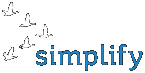You are here:  Portfolio >  Research and Development est | engOptimisation softwareOther softwareResearch and DevelopmentHomepagesAI Safety research Articles on topics about the AI alignment, AI safety related problems, the “Three Laws of Robotics”, and other proposed solutions. Completed: 2007 - presentAn optimized JavaScript implementation of the MurmurHash-3 algorithm The implementation is based on the observation that JavaScript is not limited to exactly 32-bit integers. Rather, it internally represents integers as double-precision floats. Therefore it is limited to 52-bit integers (the mantissa length). 52 bits is enough to contain 48 bits and therefore it is able to contain the result of multiplying 32-bit and 16-bit numbers without loss of significant bits. This observation enables the algorithm to be implemented so that it requires less operations to emulate multiplications of 32-bit members, as compared to an algorithm that uses multiplications of 16-bit members to emulate multiplications of 32-bit members. Also because of that, addition of 32-bit operands can be performed simpler. Completed: 24. January 2015Framework for modelling of Natural General Intelligence This project aims at creation of open source AGI (Artificial General Intelligence) through modeling of natural thinking. The framework contains working model of classical and operant conditioning, plus insight learning. The framework is flexible, not brittle. It is partially written using C++ metaprograms in such a way that it is easy to modify, add or remove model's components, without breaking the whole system or overwhelming programmers attention. Additionally it contains multiple general-purpose libraries (for example: segmented linked lists; a metaprogram for generating net-like "pipeline" structures; a substantial extension of Boost "Parameter" library; metaprograms for generating SSE vector code) - which may become separate projects in the future. One can test this framework for example connecting it with controller for SimRobot robot simulation software (SimRobot is a separate project), but other uses should be also handy without much modification. Completed: 22. May 2007Methods for calculating precise logarithm of a sum and subtraction Disclosed are methods to compute the precise value of the logarithm of a sum and the logarithm of a subtraction. A number of practical problems can result in having too big or small values in intermediate values of a calculation. Then one tries to take logarithm of these values and operate on logarithms instead. In the case: log (p • q) equals log p + log q is very easy to compute, but the problem is then to compute (or approximate) the value of log (a + b) from the value of log a and log b. Let us assume that log a and log b are known, and that we want to approximate log (a + b). Most basic solution would be calculating sum_log equals log(exp(a_log) + exp(b_log)), where a_log equals log a and b_log equals log b and therefore sum_log equals log(a + b). But the method I propose requires calling only one exp() and one log(), instead of two exp() and one log() in the basic solution. Additionally, the proposed method has the critical advantage of not overflowing in case of large numbers of a and b. Completed: Spring 2007 Order a software© Simplify | Macrotec LLC | gsm +372 57 843 938, +372 58 058 134 | e-mail: info@simplify.ee